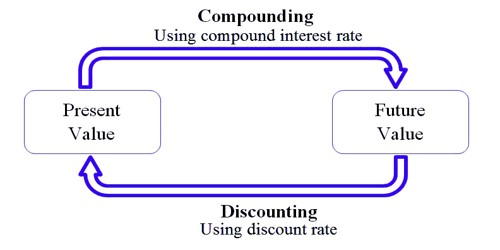# Difference between Discounting and Compounding

The process used to resolve the future value of the present investment is known as Compounding. It is the procedure of earning interest over time. And the technique used to decide the present value of future cash flows is known as Discounting. is the procedure of converting future cash flows to what its present value is.

The difference between discounting and compounding are discussed below:

Discounting:

1. Definition: Discounting is the process of finding the present value 01 future cash flow or series of cash. In other words, the present value is the current value of the future cash flows that are discounted at an appropriate interest rate.
2. Use: For calculation of resent value. What should be the amount we need to invest today, to get a specific amount in the future?
3. Amount of money: By this method, the amount of money is decreased.
4. Value of money: In lower rate present value is increased and in higher rate present value is decreased. Discounting finds the present value of some future value, using a discount rate.
5. Time Line: Time lie goes to the left side from the right-hand side.
6. Calculation: Future values are divided b interest factor.
7. Result: Present or a discounted value. Present Value Factor.

Compounding:

1. Definition: Compounding is the process of finding the future value of cash flow or a series of cash flows.
2. Use: For calculation of future value. If we invest some money today, what will be the amount we get at a future date?
3. Amount of money: Amount of money is increased. Compounding finds the future value of present value using a compound interest rate.
4. Value of money: In lower rate future value is decreased and a higher rate of future value is increased.
5. Time Line: Timeline goes to right side from left-hand side.
6. Calculation: Present value is multiplied by the interest factor.
7. Result: Future or compounded value. Future Value Factor.